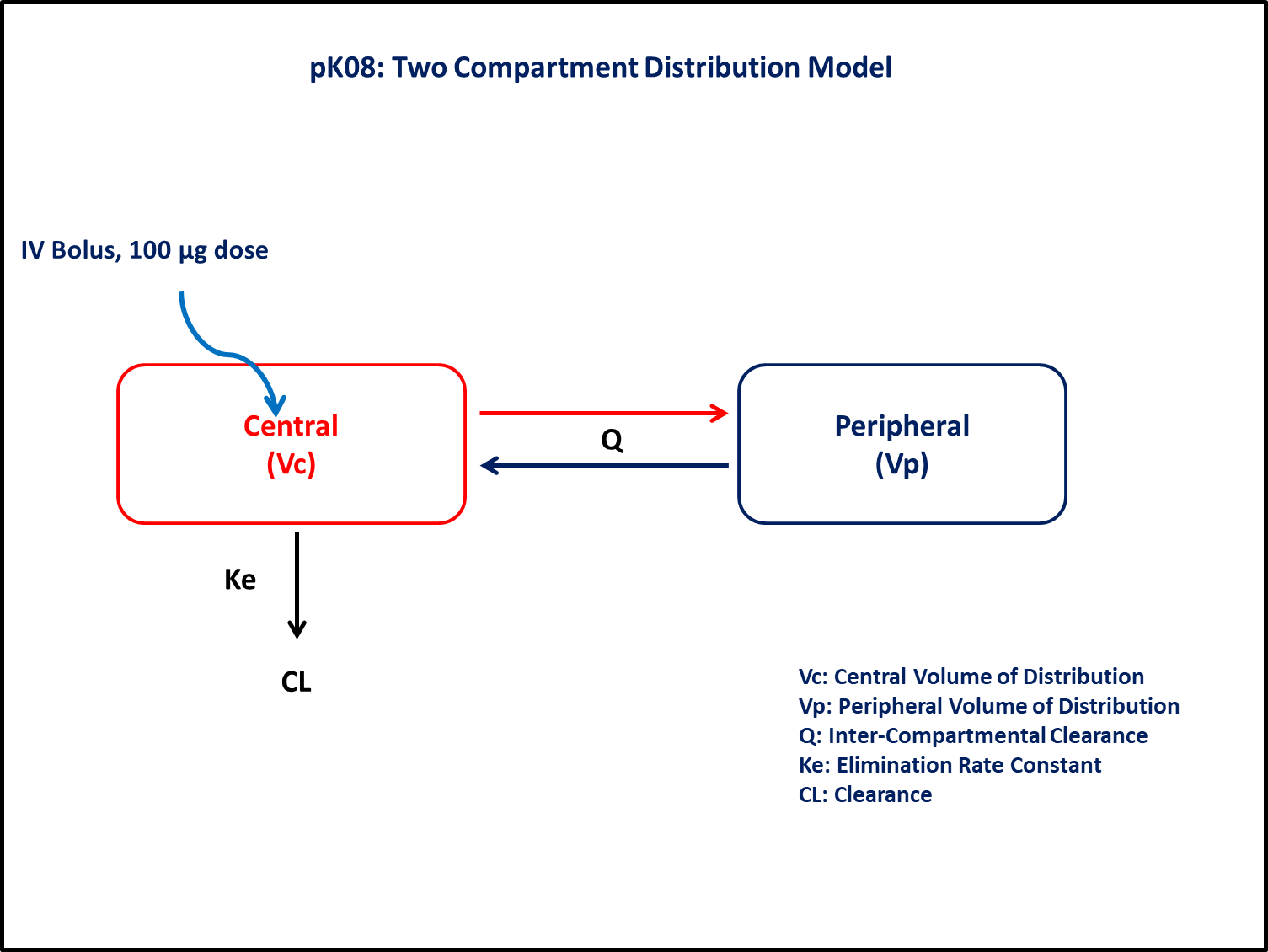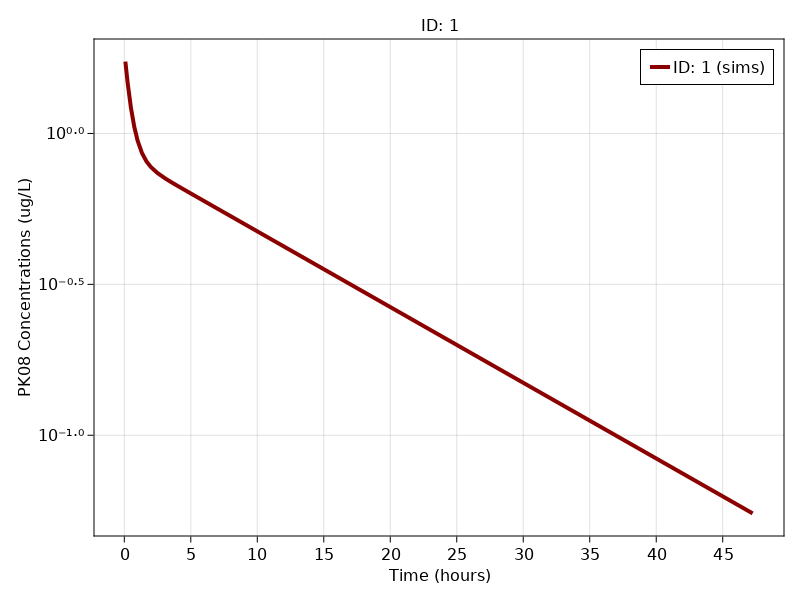# Exercise PK08 - Two-Compartment Distribution Models (Part 5 - Using Differential Equations)

### Background

• Structural model - Two compartment linear elimination with first order elimination

• Route of administration - IV bolus,

• Dosage Regimen - 100 μg IV or 0.1 mg IV

• Number of Subjects - 1### Learning Outcome

This exercise explains simulating single IV bolus dose kinetics from a two compartment model.

### Objectives

To build a two compartment model and to simulate the model for a single subject given a single IV bolus dose.

### Libraries

call the "necessary" libraries to get started.

using Random
using Pumas
using PumasUtilities
using CairoMakie


### Model

pk_08_05        = @model begin
desc = "Two Compartment Model"
timeu = u"hr"
end

@param begin
"Clearance (L/hr)"
tvcl        ∈ RealDomain(lower=0)
"Volume of Distribution (L)"
tvvc        ∈ RealDomain(lower=0)
"Intercompartmental Clearance (L/hr)"
tvq         ∈ RealDomain(lower=0)
"Peripheral Volume of Distribution (L)"
tvvp        ∈ RealDomain(lower=0)
Ω           ∈ PDiagDomain(4)
"Proportional RUV"
σ²_prop     ∈ RealDomain(lower=0)
end

@random begin
η           ~ MvNormal(Ω)
end

@pre begin
Cl          = tvcl * exp(η)
Vc          = tvvc * exp(η)
Vp          = tvvp * exp(η)
Q           = tvq * exp(η)
end

@dynamics begin
Central'    = -(Cl/Vc)*Central - (Q/Vc)*Central + (Q/Vp)*Peripheral
Peripheral' =  (Q/Vc)*Central  - (Q/Vp)*Peripheral
end

@derived begin
"""
PK08 Concentration (ug/L)
"""
cp          = @. Central/Vc
"""
PK08 Concentration (ug/L)
"""
dv          ~ @. Normal(cp, sqrt(cp^2*σ²_prop))
end
end

PumasModel
Parameters: tvcl, tvvc, tvq, tvvp, Ω, σ²_prop
Random effects: η
Covariates:
Dynamical variables: Central, Peripheral
Derived: cp, dv
Observed: cp, dv


### Parameters

• $C$L - Clearance(L/hr),

• $V$c - Volume of Central Compartment(L),

• $V$p - Volume of Peripheral Compartment(L),

• $Q$ - Inter-departmental clearance(L/hr),

• $Ω$ - Between Subject Variability,

• $σ$ - Residual error

param  = (tvcl    = 6.6,
tvvc    = 53.09,
tvvp    = 57.22,
tvq     = 51.5,
Ω       = Diagonal([0.0,0.0,0.0,0.0]),
σ²_prop = 0.047)

(tvcl = 6.6, tvvc = 53.09, tvvp = 57.22, tvq = 51.5, Ω = [0.0 0.0 0.0 0.0;
0.0 0.0 0.0 0.0; 0.0 0.0 0.0 0.0; 0.0 0.0 0.0 0.0], σ²_prop = 0.047)


### Dosage Regimen

Dosage Regimen - 100 μg or 0.1mg of IV bolus was given to the single subject.

ev1   = DosageRegimen(100, time = 0, cmt = 1, evid = 1, addl = 0, ii = 0)
sub1  = Subject(id = 1, events = ev1)

Subject
ID: 1
Events: 1


### Simulation

To simulate the plasma concentration with given observation time-points for single subject.

Random.seed!(123)
sim_s1 = simobs(pk_08_05,sub1,param,
obstimes=[0.08,0.25,0.5,0.75,1,1.33,1.67,2,2.5,3.07,3.5,4.03,5,7,11,23,29,35,47.25]);


### Visualize results

f, a, p = sim_plot(pk_08_05, [sim_s1],
observations = :cp,
color = :redsblues, linewidth = 4,
axis = (xlabel = "Time (hours)",
ylabel = "PK08 Concentrations (ug/L)",
xticks = 0:5:50, yscale = log10))
axislegend(a)
f